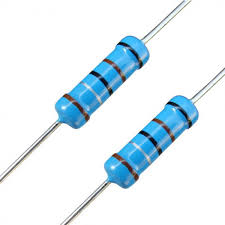# Voltage - Kirchoff laws

At a voltage of 2.5V, a current I1= 20mA flows through the resistor. What will be the voltage at the ends of the resistor when the current I2= 1,2A passes through it?

U2 =  150 V

### Step-by-step explanation:Did you find an error or inaccuracy? Feel free to write us. Thank you!

Tips for related online calculators
Need help calculating sum, simplifying, or multiplying fractions? Try our fraction calculator.